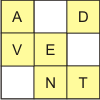Search by Topic

Resources tagged with Working systematically similar to Baravelle:

Filter by: Content type:
Age range:
Challenge level:

There are 340 results

Broad Topics > Using, Applying and Reasoning about Mathematics > Working systematicallyInstant Insanity

Age 11 to 18 Challenge Level:

Given the nets of 4 cubes with the faces coloured in 4 colours, build a tower so that on each vertical wall no colour is repeated, that is all 4 colours appear.Squares in Rectangles

Age 11 to 14 Challenge Level:

A 2 by 3 rectangle contains 8 squares and a 3 by 4 rectangle contains 20 squares. What size rectangle(s) contain(s) exactly 100 squares? Can you find them all?Tetrahedra Tester

Age 11 to 14 Challenge Level:

An irregular tetrahedron is composed of four different triangles. Can such a tetrahedron be constructed where the side lengths are 4, 5, 6, 7, 8 and 9 units of length?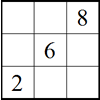Twin Corresponding Sudoku III

Age 11 to 16 Challenge Level:

Two sudokus in one. Challenge yourself to make the necessary connections.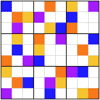Ratio Sudoku 3

Age 11 to 16 Challenge Level:

A Sudoku with clues as ratios or fractions.First Connect Three

Age 7 to 14 Challenge Level:

Add or subtract the two numbers on the spinners and try to complete a row of three. Are there some numbers that are good to aim for?LOGO Challenge - Triangles-squares-stars

Age 11 to 16 Challenge Level:

Can you recreate these designs? What are the basic units? What movement is required between each unit? Some elegant use of procedures will help - variables not essential.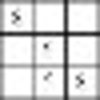Twin Corresponding Sudokus II

Age 11 to 16 Challenge Level:

Two sudokus in one. Challenge yourself to make the necessary connections.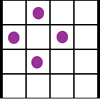Square Corners

Age 7 to 11 Challenge Level:

What is the greatest number of counters you can place on the grid below without four of them lying at the corners of a square?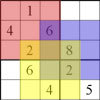Twin Corresponding Sudoku

Age 11 to 18 Challenge Level:

This sudoku requires you to have "double vision" - two Sudoku's for the price of one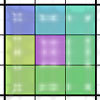Diagonal Sums Sudoku

Age 7 to 16 Challenge Level:

Solve this Sudoku puzzle whose clues are in the form of sums of the numbers which should appear in diagonal opposite cells.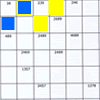Intersection Sudoku 1

Age 11 to 16 Challenge Level:

A Sudoku with a twist.Colour in the Square

Age 7 to 16 Challenge Level:

Can you put the 25 coloured tiles into the 5 x 5 square so that no column, no row and no diagonal line have tiles of the same colour in them?Age 11 to 16 Challenge Level:

Four numbers on an intersection that need to be placed in the surrounding cells. That is all you need to know to solve this sudoku.More Magic Potting Sheds

Age 11 to 14 Challenge Level:

The number of plants in Mr McGregor's magic potting shed increases overnight. He'd like to put the same number of plants in each of his gardens, planting one garden each day. How can he do it?Window Frames

Age 5 to 14 Challenge Level:

This task encourages you to investigate the number of edging pieces and panes in different sized windows.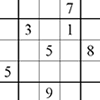Corresponding Sudokus

Age 11 to 18

This second Sudoku article discusses "Corresponding Sudokus" which are pairs of Sudokus with terms that can be matched using a substitution rule.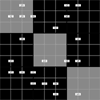Ratio Sudoku 1

Age 11 to 16 Challenge Level:

A Sudoku with clues as ratios.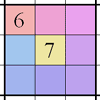Wallpaper Sudoku

Age 11 to 16 Challenge Level:

A Sudoku that uses transformations as supporting clues.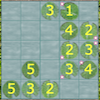Latin Lilies

Age 7 to 16 Challenge Level:

Have a go at this game which has been inspired by the Big Internet Math-Off 2019. Can you gain more columns of lily pads than your opponent?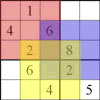Seasonal Twin Sudokus

Age 11 to 16 Challenge Level:

This pair of linked Sudokus matches letters with numbers and hides a seasonal greeting. Can you find it?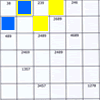Intersection Sudoku 2

Age 11 to 16 Challenge Level:

A Sudoku with a twist.Games Related to Nim

Age 5 to 16

This article for teachers describes several games, found on the site, all of which have a related structure that can be used to develop the skills of strategic planning.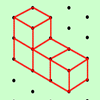The Third Dimension

Age 5 to 11 Challenge Level:

Here are four cubes joined together. How many other arrangements of four cubes can you find? Can you draw them on dotty paper?Sealed Solution

Age 7 to 11 Challenge Level:

Ten cards are put into five envelopes so that there are two cards in each envelope. The sum of the numbers inside it is written on each envelope. What numbers could be inside the envelopes?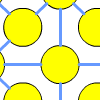One to Fifteen

Age 7 to 11 Challenge Level:

Can you put the numbers from 1 to 15 on the circles so that no consecutive numbers lie anywhere along a continuous straight line?Code Breaker

Age 7 to 11 Challenge Level:

This problem is based on a code using two different prime numbers less than 10. You'll need to multiply them together and shift the alphabet forwards by the result. Can you decipher the code?Single Track

Age 7 to 11 Challenge Level:

What is the best way to shunt these carriages so that each train can continue its journey?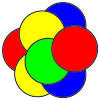Counters in the Middle

Age 7 to 11 Challenge Level:

This task depends on groups working collaboratively, discussing and reasoning to agree a final product.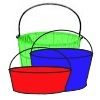More and More Buckets

Age 7 to 11 Challenge Level:

In this challenge, buckets come in five different sizes. If you choose some buckets, can you investigate the different ways in which they can be filled?Problem Solving, Using and Applying and Functional Mathematics

Age 5 to 18 Challenge Level:

Problem solving is at the heart of the NRICH site. All the problems give learners opportunities to learn, develop or use mathematical concepts and skills. Read here for more information.Sticky Numbers

Age 11 to 14 Challenge Level:

Can you arrange the numbers 1 to 17 in a row so that each adjacent pair adds up to a square number?Triangles to Tetrahedra

Age 11 to 14 Challenge Level:

Imagine you have an unlimited number of four types of triangle. How many different tetrahedra can you make?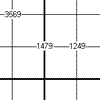Another Quadruple Clue Sudoku

Age 11 to 16 Challenge Level:

This is a variation of sudoku which contains a set of special clue-numbers. Each set of 4 small digits stands for the numbers in the four cells of the grid adjacent to this set.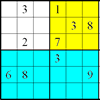Colour Islands Sudoku

Age 11 to 14 Challenge Level:

An extra constraint means this Sudoku requires you to think in diagonals as well as horizontal and vertical lines and boxes of nine.Multiply the Addition Square

Age 11 to 14 Challenge Level:

If you take a three by three square on a 1-10 addition square and multiply the diagonally opposite numbers together, what is the difference between these products. Why?Triangles All Around

Age 7 to 11 Challenge Level:

Can you find all the different triangles on these peg boards, and find their angles?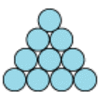Difference

Age 7 to 11 Challenge Level:

Place the numbers 1 to 10 in the circles so that each number is the difference between the two numbers just below it.Maths Trails

Age 7 to 14

The NRICH team are always looking for new ways to engage teachers and pupils in problem solving. Here we explain the thinking behind maths trails.A Square of Numbers

Age 7 to 11 Challenge Level:

Can you put the numbers 1 to 8 into the circles so that the four calculations are correct?Coded Hundred Square

Age 7 to 11 Challenge Level:

This 100 square jigsaw is written in code. It starts with 1 and ends with 100. Can you build it up?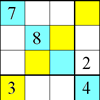Rectangle Outline Sudoku

Age 11 to 16 Challenge Level:

Each of the main diagonals of this sudoku must contain the numbers 1 to 9 and each rectangle width the numbers 1 to 4.Magic Potting Sheds

Age 11 to 14 Challenge Level:

Mr McGregor has a magic potting shed. Overnight, the number of plants in it doubles. He'd like to put the same number of plants in each of three gardens, planting one garden each day. Can he do it?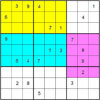Colour Islands Sudoku 2

Age 11 to 18 Challenge Level:

In this Sudoku, there are three coloured "islands" in the 9x9 grid. Within each "island" EVERY group of nine cells that form a 3x3 square must contain the numbers 1 through 9.Multiples Sudoku

Age 11 to 14 Challenge Level:

Each clue in this Sudoku is the product of the two numbers in adjacent cells.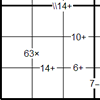Bochap Sudoku

Age 11 to 16 Challenge Level:

This Sudoku combines all four arithmetic operations.Consecutive Negative Numbers

Age 11 to 14 Challenge Level:

Do you notice anything about the solutions when you add and/or subtract consecutive negative numbers?Extra Challenges from Madras

Age 11 to 14 Challenge Level:

A few extra challenges set by some young NRICH members.Mystery Matrix

Age 7 to 11 Challenge Level:

Can you fill in this table square? The numbers 2 -12 were used to generate it with just one number used twice.